### Home > A2C > Chapter 5 > Lesson 5.2.2 > Problem5-83

5-83.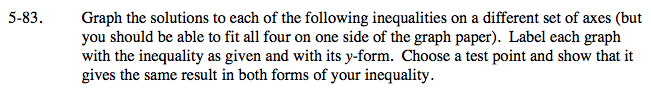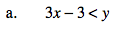y > 3x − 3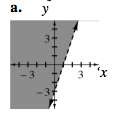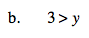y < 3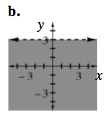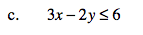Rewrite the inequality as an equation and solve for y. Graph the line.

Does the point (0, 0) make the original inequality true?

You need to decide whether to use ≥ or ≤. Since (0, 0) worked in the original inequality, it should work in the one you are writing as well. Try one symbol and test (0, 0). If it is not true, try it the other way.

$\textit{y} \ge \frac{3}{2} \textit{x} -2$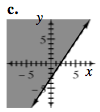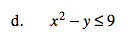Repeat the steps used in part (a): Solve for y; graph the parabola; test (0, 0); decide where to shade.

yx2 − 9